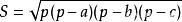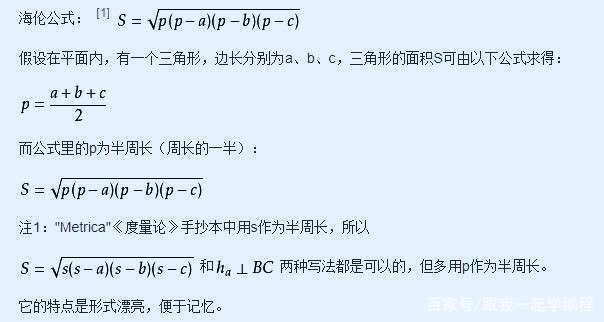# python利用海伦公式求三角形的面积

### 前言python根据三角形三条边求面积

1.三角形的三条边的符合条件

2.海伦公式求三角形面积

a = float(input('输入三角形第一边长： '))

b = float(input('输入三角形第二边长： '))

c = float(input('输入三角形第三边长： '))

while a+b<c or a+c<b or b+c<a:

print('输入的边构不成三角形，请重新输入！')

a =float(input ('输入三角形第一边长：'))

b =float(input ('输入三角形第二边长：'))

c =float(input ('输入三角形第三边长：'))

# 计算半周长

s = (a + b + c) / 2

# 计算面积

area = (s*(s-a)*(s-b)*(s-c)) ** 0.5

print('三角形面积为 %0.2f' %area)

### 前言python根据三角形三条边求面积

1.三角形的三条边的符合条件

2.海伦公式求三角形面积

a = float(input('输入三角形第一边长： '))

b = float(input('输入三角形第二边长： '))

c = float(input('输入三角形第三边长： '))

while a+b<c or a+c<b or b+c<a:

print('输入的边构不成三角形，请重新输入！')

a =float(input ('输入三角形第一边长：'))

b =float(input ('输入三角形第二边长：'))

c =float(input ('输入三角形第三边长：'))

# 计算半周长

s = (a + b + c) / 2

# 计算面积

area = (s*(s-a)*(s-b)*(s-c)) ** 0.5

print('三角形面积为 %0.2f' %area)

### 总结02-03108310-121万+
03-044629
11-301049
10-2980
11-021万+
12-061873
03-111万+
09-20
12-17175
12-163018
11-052707
04-024350
04-041037
12-11400
02-056217
03-253316
08-1576万+
©️2020 CSDN 皮肤主题: 游动-白 设计师:白松林